# Preorder Traversal of binary trees

To preorder a non-empty binary tree, below operations are preformed recursively of each node

1. visiting the root node
2. traversing the left subtree
3. traversing the right subtree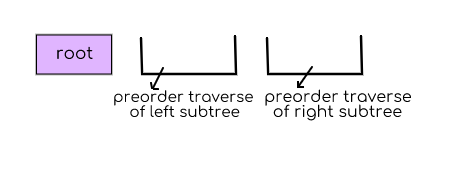the word “pre” specifies that the root node is accessed prior to any other node from the left and right subtrees

lets see one example: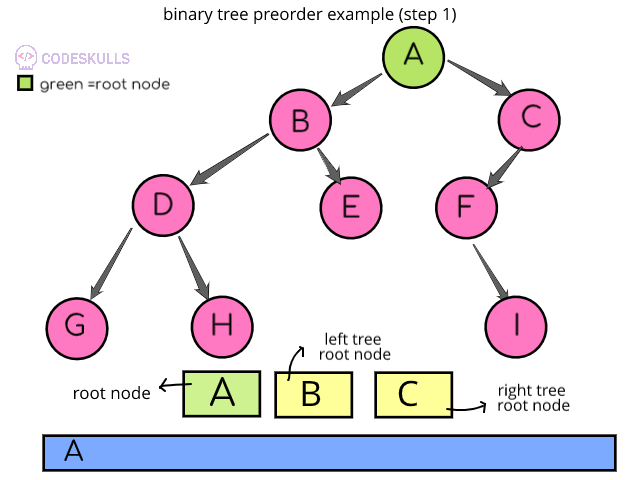step1: in the above binary tree, A is the root node so it accessed first, then in pre-order next priority will be left subtree root node B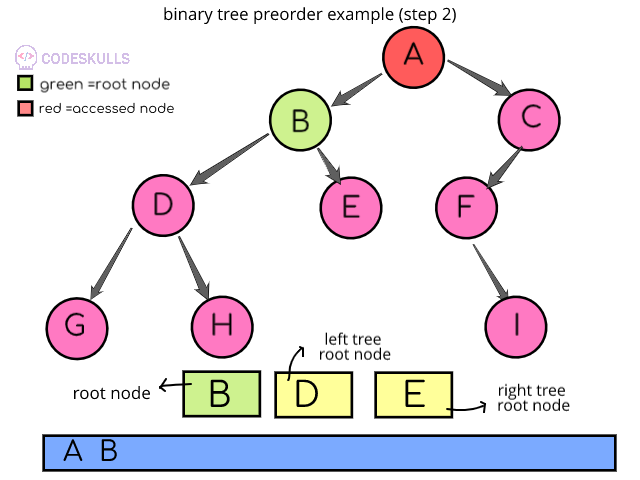step2: now, B is the root node so be will be accessed and next the left subtree root D will be accessed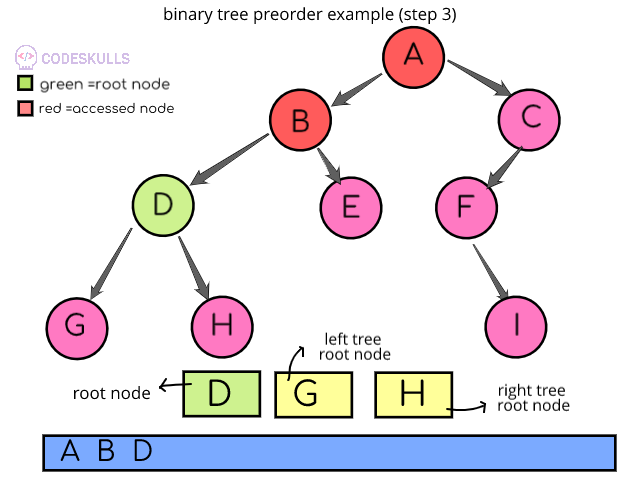step 3: now, D is the root node so be will be accessed and next the left subtree root G will be accessed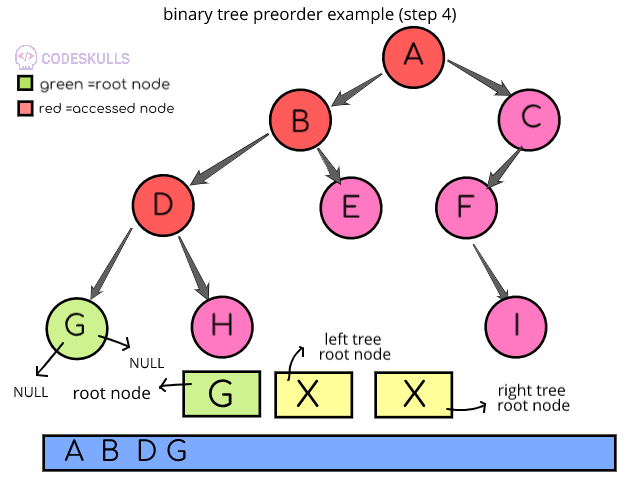step 4:G is the root node, there are no child nodes for the G node, so the next again parent node of G node, D node will be accessed to perform preorder traverse of the right subtree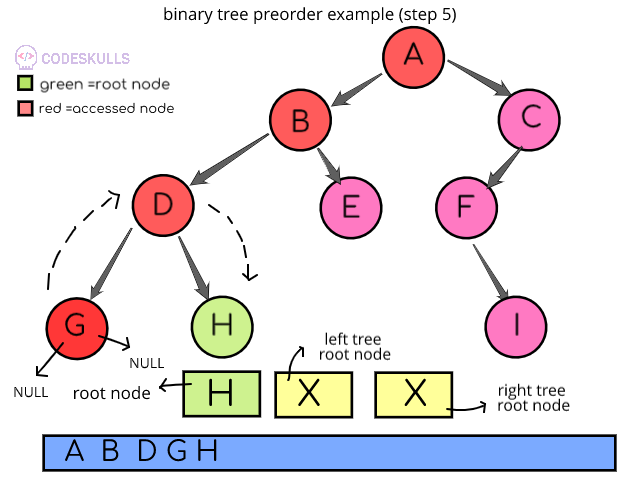step 5:after moving from node G , node D will be accessed then left subtree of node D is completed so, right subtree will be accessed, right subtree tree contains node Hstep 6:after moving from node H, then its parent node D will be accessed, now the preorder operation is done on both left and right subtree of node D,so next node B will be accessed, from the node B , right subtree node E will be accessed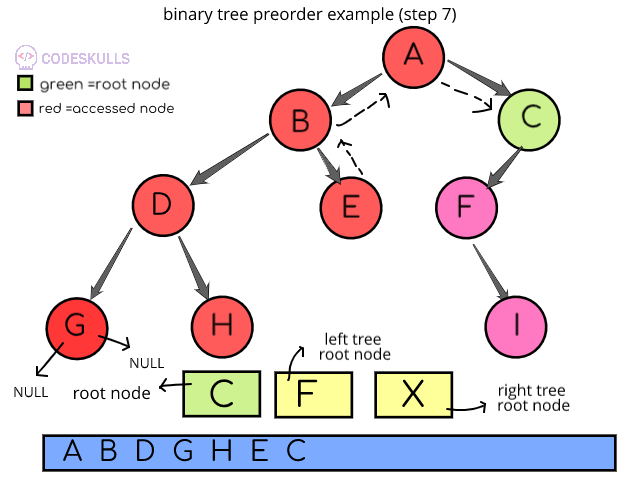step 7:after moving from node E, then its parent node B will be accessed, now the preorder operation is done on both left and right subtree of node B,so next node A will be accessed, from the node A , right subtree node C will be accessed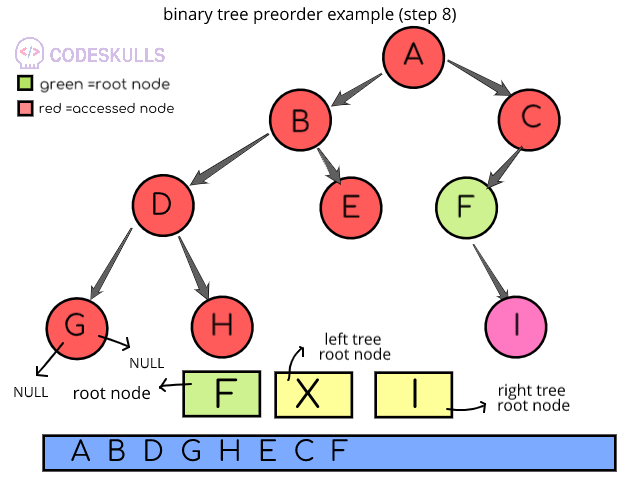step 8:from node C , left subtree root will be node F, node F will be accessed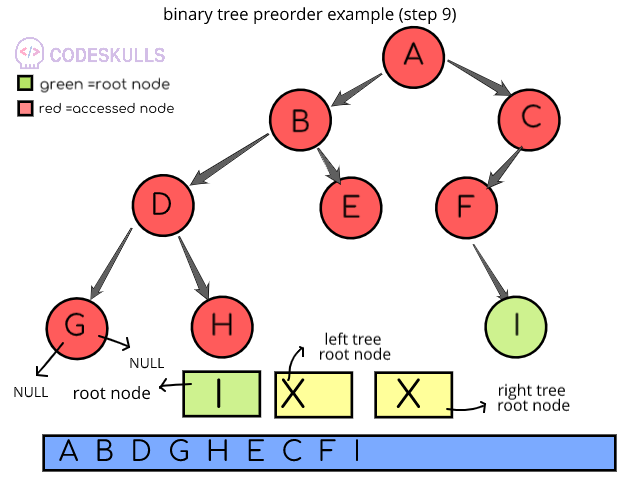step 9:there is no left subtree for node F, so right sub-tree root node I will be accessed

Last updated on by vishal devxo